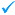150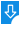10
2585

# Gpa and CGPA Calculator  Free Download

Gpa and CGPA Calculator Description:
Gpa and CGPA Calculator is an application that allows you to calculate the CGPA and GPA for any university under UGC in Bangladesh. Gpa and CGPA Calculator is developed using the Java programming language and can be run on Mac OS X, Windows and Linux.Gpa and CGPA Calculator: Gpa and CGPA Calculator for Mac, Java tool to calculate the CGPA and GPADownload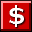Buy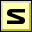Screenshot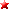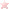Rate it
Category: Math